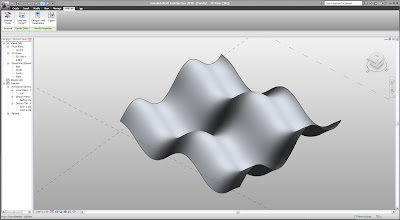## Tuesday, January 5, 2010

### Revit API: Mathematical Form-Making

In addition to my continued exploration with Grasshopper, I will begin doing posts relating to my recent interest with the Revit API.... and as my previous post suggests, these interests are not necessarily going to be "exclusive" from one another....

Below are some examples of making some parametric surfaces using a simple API script. The kinds of surfaces you are able to create is limited for two reasons:
1. There are only about 4 ways to create a surface (Loft, extrude, sweep, revolve)...
A surface from a point cloud or from 4-points would be very handy, Autodesk!
2. Revit doesn't seem to "like" self-intersecting surfaces very much... and even sometimes mistakes 'closed' shapes or shapes where edges touch for self-intersection....so no Kleins or Catalans, booo!

Wave SurfaceSample Code (Wave surface):
Dim ref_ar_ar As ReferenceArrayArray = New ReferenceArrayArray()
Dim XYZ As Autodesk.Revit.Geometry.XYZ

For u As Double = 0 To 4 * Math.PI Step Math.PI / 4
Dim rfptsarr As ReferencePointArray = New ReferencePointArray()
For v As Double = 0 To 4 * Math.PI Step Math.PI / 4

Dim refpt As Autodesk.Revit.Elements.ReferencePoint

Dim x As Double
Dim y As Double
Dim z As Double

x = 10 * u
y = 10 * v
z = 10 * Math.Cos(u) + 10 * Math.Sin(v)

XYZ = revit_app.Create.NewXYZ(x, y, z)
refpt = revit_doc.FamilyCreate.NewReferencePoint(XYZ)

rfptsarr.Append(refpt)
Next
Dim crv As CurveByPoints = revit_doc.FamilyCreate.NewCurveByPoints(rfptsarr)
Dim ref_ar As ReferenceArray = New ReferenceArray()
ref_ar.Append(crv.GeometryCurve.Reference)
ref_ar_ar.Append(ref_ar)
Next

Dim loftform As Autodesk.Revit.Elements.Form = revit_doc.FamilyCreate.NewLoftForm(True, ref_ar_ar)

Enjoy!